Revised 02/09/2020

# Geometry of a 3 Sided Bowl

This page will explore the geometry of a 3 sided bowl turned from a block of wood. Why? Knowing about its geometry allows one to plan a turning before it is placed on the lathe. This may enable the turner to make better use of the wood including the ability to make more than one item from the block.

## Part 1: The Analysis

The analysis uses some very simple math all of which taught or used in high school geometry courses. Lets look at what is used for the sake of anyone who has forgotten what they learned in their geometry and earlier math classes.

1. Square roots. For example, the square root of 9 is 3 because 3 squared is 9. We will use the notation 9 = 3. We will do the algebra for you so you can follow the work even if you have forgotten how to manipulate square roots. But if you are interested, you may recall ab = √ab, a/b = √a/√b and a2 = a. Typically we avoid writing square roots in denominator. This can be done by a technique called rationalize the denominator.
```      1/√a = (1/√a) x (√a/√a)
= √a/a```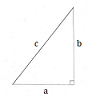2. The Pythagorean theorem. It applies to right triangles with a 90o right angle. It states that the square of the length of the long side, called the hypotenuse, equals the sum of the squares of the lengths the two shorter sides. If we use c for the length of the hypotenuse and a and b as the lengths of the other 2 sides, the mathematical notation for this rule is c2 = a2 + b2 or c = √a2 + b2.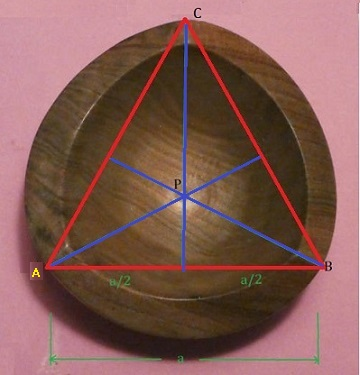3. Equilateral triangles. Equilateral triangles are triangles with 3 equal sides. If you look at the picture on the left which is the top view of a 3 sided bowl, you can see why they are relevant. We see the red lines connecting the 3 corners of the bowl form an equilateral triangle which has some very helpful properties. The corners, called vertices of the triangle are denoted by A, B, and C. The blue lines are perpendicular (right angle) to the red lines and meet at point P, the so-called center of the triangle. These lines bisect the corresponding red lines dividing them in half. The lines from P to the vertices are called circumradii. If we denote the length of the sides by a, the length of a blue line (called an altitude) is a√3/2 and the length of a circumradius (like PC) is 2/3rds of that length, namely a√3/3. One last comment. We say the equilateral triangle is inscribed in the circle formed by the outside of the bowl.

If desired, you can review the properties of equilateral triangles in web pages such as https://brilliant.org/wiki/properties-of-equilateral-triangles or even https://en.wikipedia.org/wiki/Equilateral_triangle.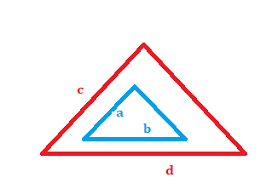4. Similar. In geometry similar objects have the same shape. One is just an enlargement of the other. In the figure on the right, the red and blue figures are similar and the linear dimensions are proportional. Thus, if a, b, c, and d are lengths of their sides, then a/b = c/d.

-------

Turning a normal 3 sided bowl is fairly a standard technique that does not require any special knowledge of its geometrical properties. But knowing some may allow advanced variations of this shape and/or more economical use of the cube allowing one to turn multiple objects from the same cube.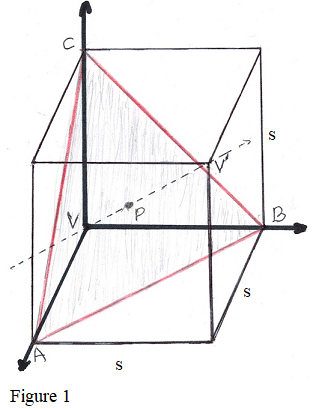Consider Figure 1 shown on the left. A cube with edges of length s is shown. Five of its eight vertices (corners) are marked V, V', A, B, and C.

We would place the cube in the lathe using the opposite vertices V and V' and the dashed line becomes the center line about which the cube is rotated. Then vertices A, B, and C are in a plane perpendicular to the center line axis of rotation. This plane is outlined in red and lightly shaded. A, B, and C are the three vertices closest to vertex (corner) V.

Using the Pythagorean theorem (c2 = a2 + b2), we can determine that the length of the diagonals such as the lines AC, AB and BC across sides of the cube have length s√2, that is, s times the square root of 2. Using the theorem a second time one can determine the diagonals between opposite vertices such as between V and V' have length s√3, that is, s times the square root of 3.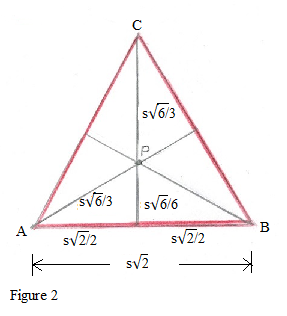Now let us assume that we cut the cube along the plane containing the vertices A, B, and C. The result is shown in Figure 2. The boundary lines are shown in red as in the previous figure. These lines are diagonals of a side of the cube and we have determined their length is s√2. Because all of its sides have equal lengths, the triangle ABC is an equilateral triangle.

One of the basic properties of equilateral triangles says that if you draw the vertical line though C, it will bisect the line AB, so each half of the base has length s√2/2.

If we draw the same lines through vertices A and B, the equilateral triangle properties say that all of the lines will meet at one point P, the "center" of the triangle. That point is significant as it is on the line that is the center of rotation for the entire blank. Applying some more well known properties of equilateral triangles, we find that the lines from point P to the vertices have length s√6/3.) (You check this out using the normal formulas for an equilateral triangle, remembering each side of this triangle has length s√2.) That means that the theoretical radius of 3 sided bowl is s√6/3 and its diameter is 2s√6/3. This ignores any rounding of the corners, sanding, or off centering when placing the cube or the parts in the lathe.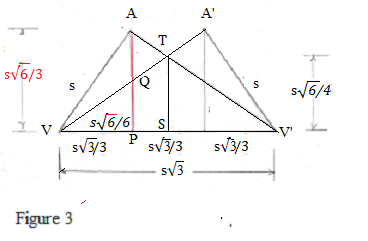Now that we have the radius and diameter of the bowl, we turn to the horizontal spacing of the vertices and Figure 3. A word about that sketch. The upside down "W" shape is top half the shape that one would see when the cube is turning on the lathe. Actually vertices A and A' never line up. If vertex A is on top, then turning the cube 60o or 180o would bring vertex A' to the position shown. Or you may think of the lines VA'and A'V' as being the inverted bottom half of the cube which is not shown in Figure 3.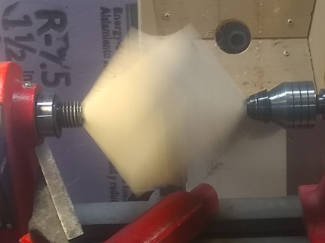A spinning cube

We know the hypotenuse of the right triangle with vertices V, P, and A has length s and the vertical line side through A has length s√6/3. Using the Pythagorean theorem again, we determine that the bottom of the triangle has length s/√3 or equivalently s√3/3. By symmetry, the other end at V' has the same length. That is neat because each is 1/3 of the length of the line between the vertices. So the distance between the vertices A and A' is also the same! That means we have the two most important measurements for the 3 sided bowl.

We will now get one more measurement that might be useful. We will determine the radius of the blank at its midpoint - its "waist". That is where the two sloped lines meet at T. We observe that the two right triangles VQP and VTS are similar - they have the same shape even though they have different sizes. The sides have the same ratio. Thus, QP/VP = TS/VS. Substituting and simplifying gives TS = s√6/4. The diameter of the blank at that point is twice that or s√6/2.

Unfortunately because of the square roots, some people may find these formulas difficult to use. An Excel like spreadsheet in Dimensions of 3 Sided Bowl provides a convenient table of calculations for various sized cubes.

Go to top of page

## Part 2: Using the Analysis

We have three examples, showing how the formulas in Part 1 can be used to help by showing the feasibility of turning 3 sided bowls in a given cube and how the part or parts can be arranged. In each case, multiple items are turned from a single cube of wood.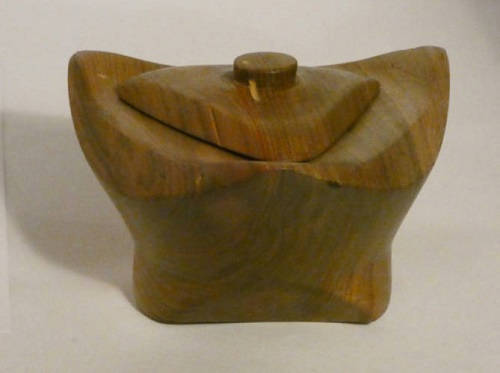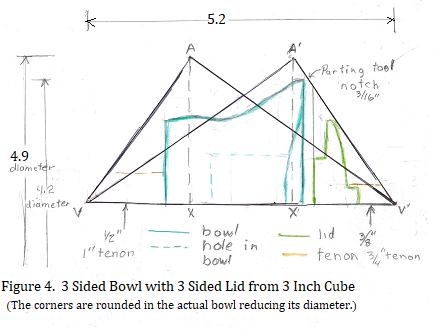Example 1: This is the example that motivated the study of the geometry of 3 sided bowls. Before starting on this bowl, I wanted to know if it was indeed possible to turn a bowl and lid from the same cube, and if so, how to organize the parts. An additional problem was the available cube was only 3 inches on each side. The formulas for the bowl length, vertex spacing and radius of the points allowed me to make a full sized drawing like the one shown in Figure 3 and graphically answer those questions. Figure 4 shows the top half of the block with bowl turned on its side as it would be in the lathe. I found that I could use 1 inch diameter (1/2 inch radius) tenon for the bowl and a 3/4 inch diameter (3/8 inch radius) tenon for the lid. Fortunately, I have a set of #1 jaws for my 4-jaw chuck because it would not have been possible to turn the bowl in such a small cube using the more standard #2 jaws. The #1 jaws can grip the small diameter tenons that were used. The tenons are rather long to help prevent the bowl or lid from flying out of the jaws. The lid handle was turned from the lid's tenon after the lid was pulled part way out of the jaws. The top fits into a 1-1/2 inch hole drilled with a sequence of forstner bits.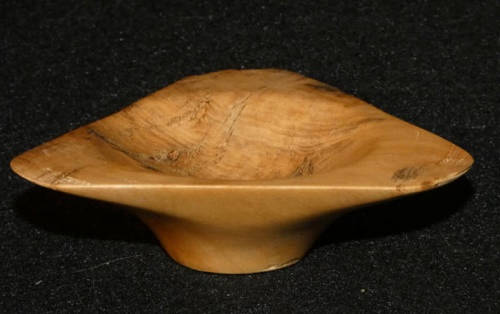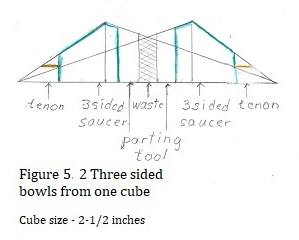Example 2: Another question was "Is it possible to turn 2 bowls from 1 cube?" It turns out that it is possible although the resulting bowls are so shallow, it might be better to call them "saucers". Figure 5 shows the 2 saucers facing each other. The layout includes notches for the parting tool used to separate the bowls. Prototypes were turned from a very small cube about 2-1/2 inches on a side. The Prototypes proved the possibility although I broke a corner off the second bowl and it turned out to be circular bowl instead. Both bowls are less than 1 inch in height.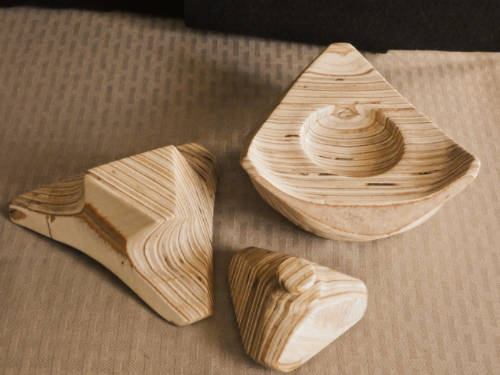Figure 6. Two bowls and a lid from one 4 inch cube

Example 3: While working on the analytics of 3 sided bowls, it occurred to me that it would be possible to use an alternate arrangement of the bowls and even include a lid for the one of the bowls. As shown in Figure 6, the two bowls are oriented in the same direction instead of facing each other. The drawings showed that at least a 4 inch cube would be needed. The two bowls are not identical. It is doubtful that one could turn this arrangement without planning using the analytical formulas. Getting 3 items from 1 cube is pretty economical use of the wood.

Update: I hadn't had time to try this example when this page was originally written. Now I have and the result is shown above. The pieces were turned from a 4-1/2 inch cube. Actually this was a double experiment. It was turned from a cube constructed of pieces of 1/4 inch plywood underlayment glued together. There was some problems with the plywood delaminating. If you look carefully, you can see one corner of the left piece in the picture (which was the right hand piece in Fig. 6) is missing. I would probably try to use better plywood in the future. I didn't follow the plan exactly. But it shows that the idea would actually work. Moreover, the shallower bowls are easier on one's fingers during the turning and sanding process than a deeper, standard 3-corner bowl. The lid looks better than the bowl used in Example 1. Another picture of the bowl with one piece used as a stand is shown in this picture.

Hint to anyone who tries this. When turning 3 cornered bowls form a cube made of plywood, significant and parts of the top and bottom face may show up in the bowls. This was particularly true in this 3 part set as can be seen in the above picture. Make sure the top and bottom are nice before gluing. Stack the plywood pieces to see how high the cube will be and cut the squares a little larger than that height. After gluing you will have to trim the sides but hopefully not the top and bottom to make a cube. Sand the sides to make them smooth before turning. Sanding the surfaces before turning is much easier than sanding the flat surfaces after turning.

Go to top of page

## Part 3: Determining the Diameter of a Completed 3 Sided Bowl

Determining the diameter of completed 3 sided bowl is rather difficult because it cannot be easily measured. Moreover, that diameter often cannot be determined theoretically because of breakage of a point or rounding of those points during turning and/or sanding process. However, assuming that the distances between the points is equal, they still form an equilateral triangle. Hence the ratios shown in Figure 2 are still valid even though the value of s may not be. Recall that in Figure 2, the distance PC was called the circumradius which is half the diameter of a circle circumscribed about the triangle. Observe

```   diameter of a completed bowl  = 2 times the circumradius
= 2(s√6/3)
= 2s√6/3```

Figure 2 shows the distance between the points of the bowl is s√2.

```   diameter of a completed bowl   2s√6/3
---------------------------- = ------
distance between the points     s√2```

Solving for the diameter and simplifying the fraction and square roots, we see

```   diameter of a completed bowl  = 2√3/3 times the distance between the points
= 1.1547 times the distance between the points```

If the points are rounded, one can still determine the best center of the rounded corner and use this formula.

Go to top of page

## Part 4: (Optional) 3 Sided Bowl Angles

This section discusses some additional properties of 3 side bowls - their angles. These results might be useful when setting a cube to be turned or may be of interest to those who are just interested in the bowl's geometry. We will be using some trigonometry - namely the tangent function. When I was in high school many years ago, that topic was covered in a more advanced high school course but it is often covered at the end of a geometry course now. So we are not really violating the statement in Part 1 that claimed that all the math used in this page is normally covered or used in high school geometry. The following are two more basic geometric principles that will be needed.

1. Complimentary angles. Two angles are complimentary if the measures of those angles add up to 90o. Figure 7a below shows the most common example. Angle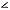BAC is a right angle and anglesBAD andDAC are complimentary. Figure 7b illustrates the second most common example of complimentary angles assuming the angle at A is a right angle (90o). It is known that the measures all three angles in a triangles in triangle add up to 180o. That means that the measures of angles at B and C add up to 90o and the angles are complementary.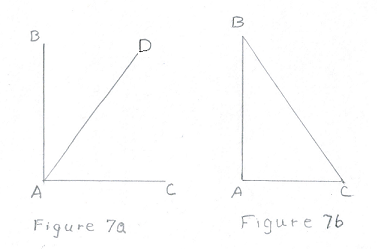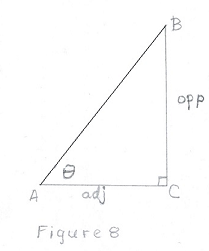2. Tangents. Note: Greek letters such as α, β, and θ are normally used for angles in trig instead of multi character names likeBAC orA.

Consider the triangle in Figure 8. The tangent of an angle θ, commonly written tan θ; is defined as the ratio of the length of the opposite side to the length of the adjacent side. That is,
`          tan θ = opp/adj`
In this paper we will know the lengths of opp and adj sides and will want to determine the angle θ. This is done with the arctangent function commonly written as arctan or
tan-1. Thus,
`           tan-1(opp/adj) = θ `
For example, suppose angle θ = 53.31o
`    tan θ = tan 53.31o = 1.5`
The sides opp and adj might be 1.5 and 1, 3 and 2, or even 300 and 200, for example. And
```         tan-1 1.5 =  tan-1 (3/2) = tan-1 (300/200) =  53.31o
```
If you have a scientific calculator and it is in the degree mode, you would generally press the button to calculate the tangent of an angle and a button such as or followed by the button in order to calculate an arctan, the angle corresponding to the given ratio.

Now that we have the basic math needed, we can determine the important angles in a 3-sided bowl.

------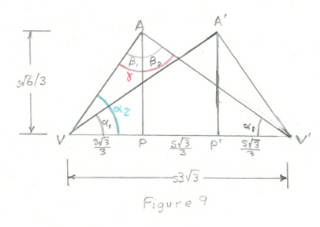We want to find α1, α2 and γ. By looking at the figure, we observe that by symmetry the two angles labeled α1 are equal justifying the labeling. Lets first look at α2. Triangle VAP is a right triangle so we observe that

```       tan α2  = AP/VP
= (s√6/3)/(s√3/3)
= √2 = 1.4142135
```

after cancelling, rationalizing the denominator, and using some properties of square roots. We find

```        α2 = tan-1 1.4142135
```

and by using scientific calculator, we find

```        α2 = 54.74o
```

The calculation of α1 is almost the same except the denominator of the fraction is twice as big.

```       tan α1  = A'P'/VP'
= (s√6/3)/(2s√3/3)
= √2/2 = 0.7071067
```

Using tan-1, we determine

```             α1 = 35.26o
```

If we add the values for α2 and α1, we find the sum is 90o and the angles are complementary!

Now that we have α2 and α1, we can find β1 and β2 easily. Recall that triangle VAP is a right triangle so α2 and β1 are complimentary and their measures add to 90o. Likewise, triangle V'AP is also a right triangle so α1 and β2 are also complimentary. Thus,

```
α2 + β1 = 90o            α1 + β2 = 90o
```

Solving for the βs, we obtain β1 = 90o - α1 = 35.26o,     β2 = 90o - α2 = 54.74o.

Again, these angles add to 90o and are also complementary and together from a right angle. So γ = 90o. Thus the angle at the point of the 3 sided bowl is a right angle (assuming the turning and sanding process has not changed the angle). The angle is a right angle of the original cube.

Go to top of page

## Part 5: Using the Angle Analysisto make a jig to cut corners from the cube.

(I plan to replace the photos in this part with ones that show a cube cut from a nicer piece of wood.)

If the math is over your, you can check out how to construct and use a jig if you click here.The α and β angles found in the previous section, have an application that might be helpful to some turners. Normally, when turning a 3 sided bowl, the cube's opposites corners are placed in the lathe without any special attachments to center the cube. But a few turners may want to turn it on centers using a drive center and 60 degree live center like they would use to turn a spindle as shown on the right. In order to use these attachments, one needs to cut off two corners of the cube, perpendicular to the axis of rotation. But cutting those corners off precisely is quite difficult. In this section, we will discuss a way of doing this taking advantage of the values found for those angles in the previous section.

In http://www.armadillowoodworks.com/pdfs/three_sided_bowl.pdf, Craig Timmerman pictures a jig he uses with a band saw to cut off corners of cubes but unfortunately, gives no details on how it is built and used. Fortunately, we can use the angles found in the previous section to build and use a different but simple jig to cut corners of a cube on a table saw. The jig could be adapted to be used on a band saw or even could be adapted to build a miter box and cut the corners by hand. First a look at the geometry.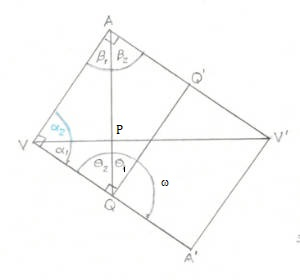Figure 10

Now, lets look at Figure 10. There are a couple of ways to interpret this figure. The first is that it is similar to Figure 9 except that vertex A' has been rotated 180o down into its actual position. That means angle α1 points downwards instead of upwards. A new construction line QQ' has been added. Right triangle marks have been added at vertices V, A, and Q and new angles θ1, θ2 and ω have been specified.

Our goal is to determine angle θ1 which turns out to be most useful with the jig. In Part 4, the lines AP and AQ were drawn perpendicular to line VV'. Also recall, we showed that α2 and α1 are complimentary. That is, their sum adds to 90o justifying the right angle mark at vertex V. Likewise, β1 and β2 are complimentary and vertex A is also a right angle. We also showed α2 = β2 and α1 = β1.

Because α1 = β1, right triangles VPA and QPV are similar triangles. This means θ2 = α2. The line QQ' was constructed perpendicular to VA' so θ1 and θ2 are also complimentary, their sum adds to 90o so ω is also a right angle.

This means that

```      θ1 = β1 = α1
= 35.26o.
```

Now lets look at figure 10 in second way. Set the cube so that vertex V is the bottom, left, front corner of the cube and V' is its top, right, back corner. Vertex A' would be on the bottom below vertex V'. Cut the cube diagonally along the plane formed by vertices V, A' and V' which is perpendicular to the bottom and at a 45o to the front of the cube. The result would be what we see in Figure 10 although Figure 10 would have to be rotated counter clockwise by an angle of α1.

Set the half cube on a table saw, perpendicular to the blade. If we tilt the blade by the angle θ1 = 35.26o we see that it could cut a corner of cube at an appropriate angle. What we need is to hold the whole cube at the 45o angle.

### The jig

Even if the above analysis is over your head, you should be able to follow the construction of the jig to cut the corners at the 45o angles.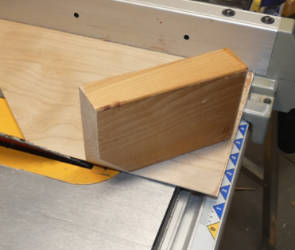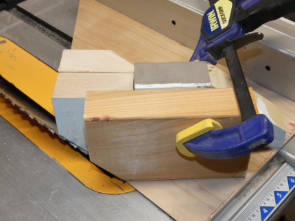A simple jig that does this shown in the left hand picture above. Piece of 2 by 4 has been fastened to a thin sheet of plywood panel at a 45o angle to the right side. The right side is pushed along a rip fence. The panel and part of the 2 by 4 was cut about 8-1/2 inches wide with the blade set at a set at a 35.26o angle. The corners are cut with the blade at the same angle. The right hand picture shows the jig in use. (One corner of the cube has already been cut off.) The cube is placed in the jig as shown and held in place by a second small piece of 2 by 4 clamped to the longer fixed piece at location determined by the size of the cut corner and pushed pass the turning blade.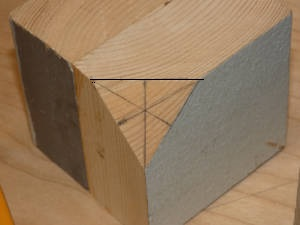The result is shown on the right. A corner was cut off. The corner has the shape of an equilateral triangle which is accurate to best that can be measured. The photo also shows a way to find the center of the triangle. The center of each side was marked and a line drawn to the opposite corner. The lines meet at the center point.

Notes:

1. The width of the bottom panel was designed to allow a 6 inch cube. However, if one really plans to use cubes bigger than about 4-1/2 inches, consider using 2 by 6 pieces instead of 2 by 4 pieces and a wider bottom board to simplify the clamping of larger blocks.
2. The fixed 2 by 4 was cut at the angle shown to provide better support for the cube as it is cut and also to provide a crude check that the blade is angled correctly.
3. If a band saw is used, the same jig can be used but one would tilt the table instead of the blade. Some way of supporting the jig such as a rip fence would be needed to keep the jig from sliding off the table.
4. If a band saw was used, the cuts were accurate enough and if planning as in Part 2 was used, then the cube could be cut with the band saw in advance instead of having to use a parting tool as suggested in that part.
5. If one wants to cut the corners by hand, one could build a specialized miter box that would hold the cube at a 45o. A slot would be needed to cut the cube at the required angle.
Go to top of page
 Dimensions of 3 Sided Bowl Length of side Length of diagonal Length between vertical points Bowl radius* Waist radius** Bowl diameter * Waist diameter** 2 3.46 1.15 1.63 1.22 3.27 2.45 2.25 3.90 1.30 1.84 1.38 3.67 2.76 2.5 4.33 1.44 2.04 1.53 4.08 3.06 2.75 4.76 1.59 2.25 1.68 4.49 3.37 3 5.20 1.73 2.45 1.84 4.90 3.67 3.25 5.63 1.88 2.65 1.99 5.31 3.98 3.5 6.06 2.02 2.86 2.14 5.72 4.29 3.75 6.50 2.17 3.06 2.30 6.12 4.59 4 6.93 2.31 3.27 2.45 6.53 4.90 4.25 7.36 2.45 3.47 2.60 6.94 5.21 4.5 7.79 2.60 3.67 2.76 7.35 5.51 4.75 8.23 2.74 3.88 2.91 7.76 5.82 5 8.66 2.89 4.08 3.06 8.16 6.12 5.25 9.09 3.03 4.29 3.21 8.57 6.43 5.5 9.53 3.18 4.49 3.37 8.98 6.74 5.75 9.96 3.32 4.69 3.52 9.39 7.04 6 10.39 3.46 4.90 3.67 9.80 7.35

 Item Formula Comment Length of side s Length of side of cube, VA Length between vertical points s√3/3 Length of AA' 1/3 of length of diagonal VV' in diagram Bowl radius* s√6/3 PA in diagram Bowl diameter* 2s√6/3 Double of bowl radius Waist radius** s√6/4 ST in diagram Waist diameter** s√6/2 Double of waist radius

* The Bowl radius and diameter are the theoretical diameter. In practice, because of sanding, rounding corners, and other factors, it will always be smaller.

** The waist radius and diameter are the diameter in the middle where the left and right slopes meet at point T.Go to top of page

Maintained By: James Brink (brinkje@plu.edu).